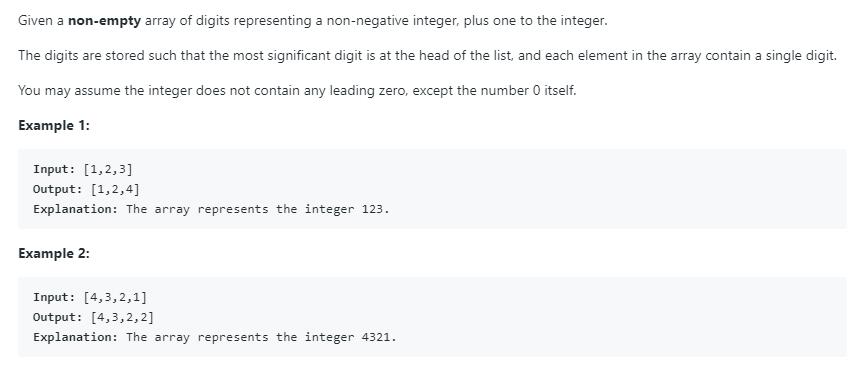# 题目描述（简单难度）# 解法一 递归

public int[] plusOne(int[] digits) {
return plusOneAtIndex(digits, digits.length - 1);
}

private int[] plusOneAtIndex(int[] digits, int index) {
//说明每一位都是 9
if (index < 0) {
//新建一个更大的数组，最高位赋值为 1
int[] ans = new int[digits.length + 1];
ans = 1;
//其他位赋值为 0，因为 java 里默认是 0，所以不需要管了
return ans;
}
//如果当前位小于 9，直接加 1 返回
if (digits[index] < 9) {
digits[index] += 1;
return digits;
}

//否则的话当前位置为 0,
digits[index] = 0;
//考虑给前一位加 1
return plusOneAtIndex(digits, index - 1);

}


# 解法二 迭代

public int[] plusOne(int[] digits) {
//从最低位遍历
for (int i = digits.length - 1; i >= 0; i--) {
//小于 9 的话，直接加 1，结束循环
if (digits[i] < 9) {
digits[i] += 1;
break;
}
//否则的话置为 0
digits[i] = 0;
}
//最高位如果置为 0 了，说明最高位产生了进位
if (digits == 0) {
int[] ans = new int[digits.length + 1];
ans = 1;
digits = ans;
}
return digits;
}